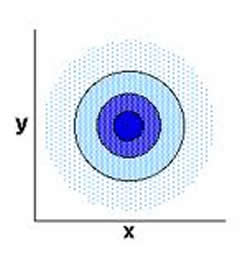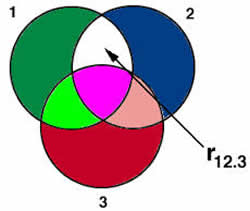Difference between Bivariate and Partial Correlation

Key Difference: The bivariate correlation is to describe the measurement of the relationship between two linear variables. On the other hand, partial correlation is to describe the measurement of two variables after allowing for the effect to third or other variables.The bivariate correlation and partial correlation are the two types of correlations in statistics. Correlation is the concept that describes the relationship between two variables. The variables are dependent on each other. Thus, the bivariate correlation and partial correlation are the tools of measurement that have a certain kind of dependency on variables.

A bivariate correlation is used in order to see if the variables are related to each other or not. It usually measures how the variables change together at the same time. The purpose of a bivariate examination is to analyze the multiple variables simultaneously. The analysis is to measure linear relationship between the two variables.Partial correlation is the correlation between two variables after allowing for the effect of other variables. They measure the correlation between two variables, but eliminate the effect of the third variable. It is best used in multiple regression. Partial correlation collects variables and it is useful for uncovering false relationships and detecting hidden relationships.

Comparison between Bivariate Correlation and Partial Correlation:

 Bivariate Correlation Partial Correlation Definition A bivariate correlation is used to measure if the two variables are related to each other or not. Partial correlation is used to measure the relation after controlling other variables (third variable). Measures It measures or analyses two variables. It measures the degree of other variables. Variables Often denoted as X and Y Two random variables, like X and Y, X and Z or Y and Z Symbol Pearson’s ‘r’ (R) rYX.W Used to obtain Used to obtain correlation coefficient that describes the measure of the relationship between two linear variables. Used to obtain correlation coefficients after controlling for one or more variables.

Image Courtesy: people.wku.edu, philender.com